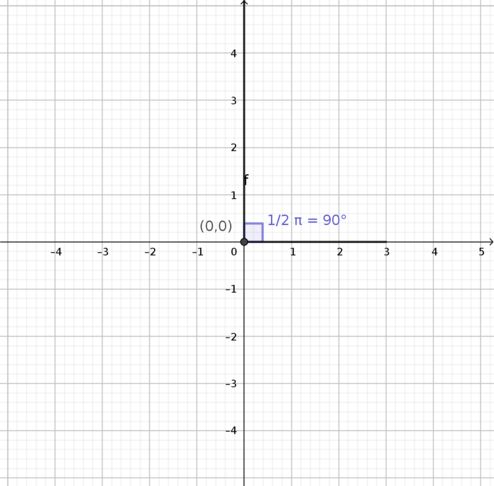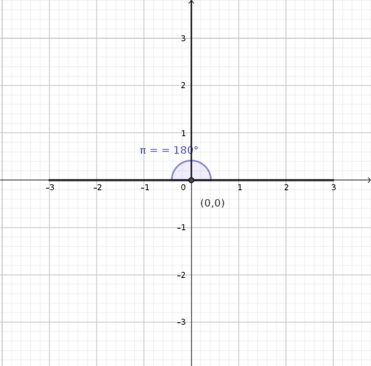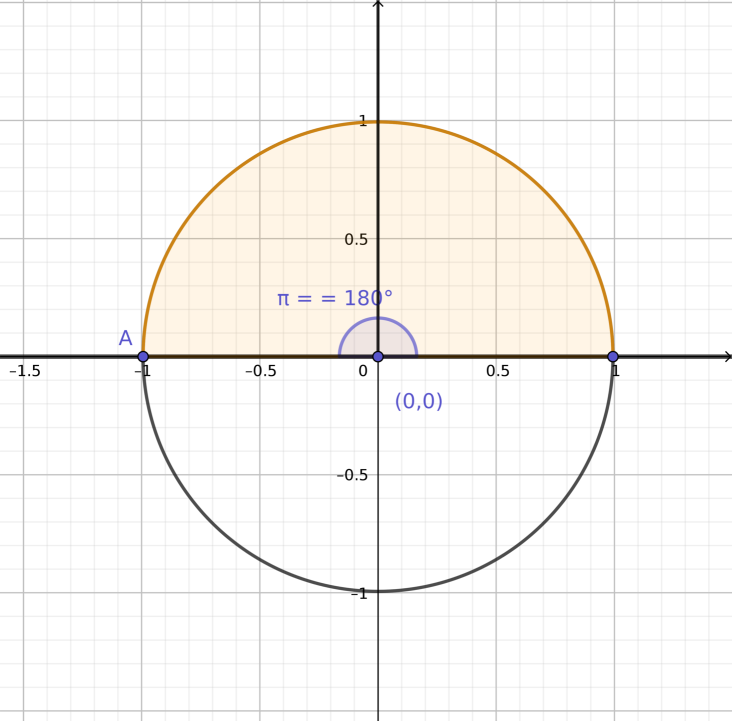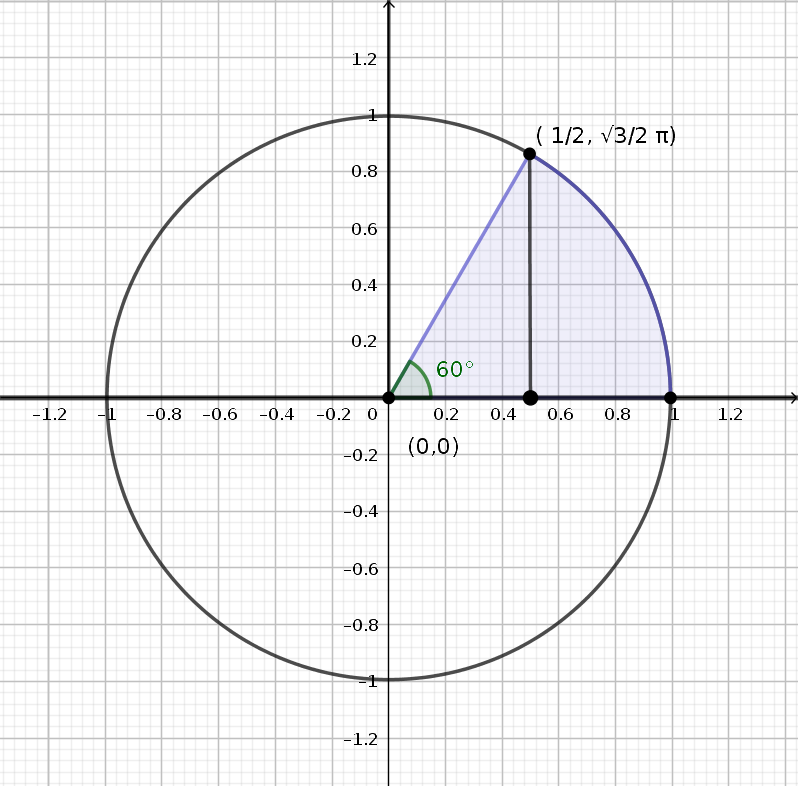# Euler

• How does Euler's identity work?

• @TSS-Graviser Oh, cool! I'm glad you asked! Euler's Identity is the inspiration for the name of Expii, the social enterprise Prof. Loh founded in order to popularize math and make education freely available to anyone.

$$e^{\pi i} = -1$$

It's been called "The most beautiful equation in math," and you can Prof. Loh talking about it in this video!

Why is it so great? Because it combines three of the fundamental constants (which is a fancy name for number, because it always has the same value, unlike a variable, which can change). These three constants are $$e, \pi,$$ and $$i.$$ Who would have thought that you could put these seemingly different constants together and get a whole number? For one thing, $$e$$ and $$\pi$$ are irrational, meaning they have an infinite number of digits in their decimal representations, and $$i$$ is an imaginary number. Somehow, $$e^{\pi i} = -1.$$

There's a lot of math that goes into this little equation. Remember the circumference of a circle with radius of $$1?$$ The circumference of a circle is $$2 \times \text{ radius } \times \pi,$$ so the circumference is $$2 \pi.$$ We can express an angle in terms of how much of a circumference a clock hand starting from the x-axis and sweeping through would cover.

For example, a right angle would sweep through one-fourth of a circle, which would be $$\frac{1}{4} \times 2 \pi,$$ or $$\frac{\pi}{2}:$$And a $$180^{\circ}$$ angle would sweep through half of a circle, which would be $$\frac{1}{2} \times 2 \pi,$$ which equals $$\pi.$$Even though it doesn't seem like it, $$\pi$$ is actually an angle! It takes a bit of getting used to, but eventually it will seem natural to think of angles in terms of $$\pi$$ instead of degrees.

The next idea you need to know in order to understand Euler's Identity is that there is a different way of drawing points on a coordinate plane. If you've heard of coordinates like $$(x,y) = (2, 3),$$ then you have heard of the first type of coordinate system, called the Cartesian system. There's another system, the complex plane. The word "complex" means that we can include imaginary numbers. What are imaginary numbers? Numbers that include $$i!$$

When you graph $$(2,3),$$ you are sort of graphing two numbers, $$2$$ and $$3.$$ On the other hand, in the complex plane, the "x" coordinate and the "y" coordinate are both part of the same number; it's just that the "y" coordinate reflects the imaginary part of the number. You write the real part first, and add the imaginary part. For example, the number $$1$$ as a complex number is

$$1 + 0i$$

and would be graphed as the blue dot sitting on the point $$1$$ along the x-axis.

Also, the number $$i$$ would equal

$$0 + i$$

and would be graphed as the point $$A$$ sitting on the blue dot at $$-1$$ on the x-axis.Now, here's the cool part: you can pretend every point is sitting on a clock hand. This is why I drew a circle above, to show the path of the tip of the clock hand. Instead of using the $$(x,y)$$ form of describing a coordinate point, you can describe a point by just giving the angle of the clock hand from the x-axis in addition to the length of the clock hand.

In this way,

$$r e^{\text{angle} \times i}$$

where $$r$$ is the length of the clock hand, and the angle is in terms of $$\pi.$$ But in our case, the length of the clock hand is $$1,$$ so $$r = 1,$$ and we just have

$$e^{\text{angle} \times i}$$

For example, the point marked $$(\frac{\pi}{2}, \frac{\sqrt{3}\pi}{2})$$ in the picture below is equal to

$$e^{\frac{\pi}{3}i}$$

because the angle $$60^{\circ}$$ is the same as $$\frac{1}{6}$$ of the whole circumference, or $$\frac{\pi}{3}.$$ We can also write this as

$$\frac{1}{2} + \frac{\sqrt{3}}{2} i$$Now we can do Euler's Identity!

If we have

$$e^{\pi i}$$

this means our angle is $$\pi,$$ which is half of a circumference, and so it is the same as $$180^{\circ}.$$ This gives us the point $$A$$ in the diagram below:This point is sitting on the x-axis, so it has no imaginary part! Since there is no number multiplied to the front of the $$e,$$ the length of the clock hand is $$1,$$ so our point is $$-1.$$

That's why

$$e^{\pi i} = -1$$• Why negative 1 and not just 1?

• @TSS-Graviser It's $$-1$$ because the point in question is point $$A,$$ which is at $$(-1,0).$$ Why does $$e^{\pi i}$$ give us this point? Because $$\pi$$ corresponds to the angle $$180^{\circ}$$ in the picture!

• Another cool application of Euler's formula is taking powers of complex numbers;

For example if you had a complex number like (sqrt(3)/2 + 1/2 i)^20 you could easily figure this out with Euler's formula

(also a great way to farm views on youtube jkjk)